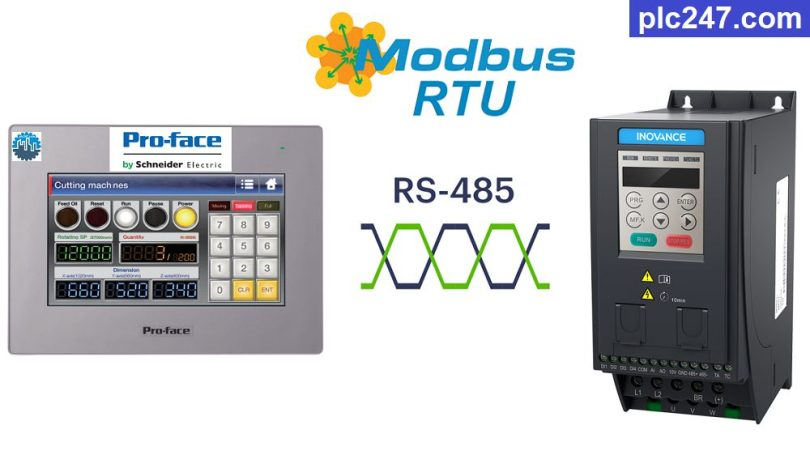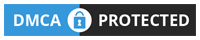# HMI Proface “Modbus RTU” Inovance MD200 TutorialWritten by

Hello friends!
In the previous article, plc247.com showed you how to use a number of different HMIs to control the Inovance MD200 inverter through Modbus RTU, today I continue to guide you to use HMI Proface to control it.

The HMI model used is Proface GP4402WW (Other Proface models have RS485 ports to do the same), plc247.com will guide you to control the Inovance MD200 inverter in the simplest and easiest way to understand.

#### Proface HMI & Inovance MD200 “Wiring Diagram”

+ Below is the RS485 communication connection diagram between HMI Proface GP4402WW and Inovance MD200 VFD

#### Inovance MD200 Modbus Parameters Setting

Below are the communication parameters that need to be installed for the Inovance MD200 inverter so that we can control and monitor it through Modbus RTU.

##### Note: Proface GP4402WW Holding Registers Address = “VFD Address (Dec) + 400001“

>>> VFD Control Address = 2000 (hex) = 8192 (dec) + 400001 = 408193 (dec)

• Set Word 8193 = 1 >>> Forward Run
• Set Word 8193 = 2 >>> Reverse Run
• Set Word 8193 = 6 >>> Stop

>>> Set Frequency Address = 1000 (hex) = 4096 (dec) + 400001 = 404097 (dec)
>>> Frequency Reference = (0 to 10000 dec) ~ (0 to VFD Max Frequency)

+ Output Frequency Address = 1001 (hex) = 4097 (dec) + 400001 = 404098 (dec)
Output Voltage Address = 1003 (hex) = 4099 (dec) + 400001 = 404100 (dec)
Output Current Address = 1004 (hex) = 4100 (dec) + 400001 = 404101 (dec)

#### HMI Proface “Modbus RTU” Configuration

As we have set the Modbus communication parameters for the Inovance MD200 inverter, the same is set up for the HMI Proface.

### HMI Modbus Programming

+ Main Page Design

+ Frequency Setting

+ Forward Run Command

+ Reverse Run Command

+ Stop Command

+ Output Frequency Monitoring

+ Output Voltage Monitoring

+ Output Current Monitoring

### Project Video Tutorial

======

###### References